# SciRealm RocksJoin the geocaching quest...Send an email with picture of the SciRealm Rock that you found and I will add your info to the list!please email John.SiteMap of SciRealm | About John | John's Resume/C.V./Bio | Send email to John John's Science, Math, Philosophy Stuff: The Science Realm: John's Virtual Sci-Tech Universe | 4-Vectors | Ambigrams | Antipodes | Covert Ops Fragments | Cyrillic Projector | Forced Induction (Sums Of Powers Of Integers) | Fractal Tree | Frontiers | IFS Fractals (Assembly) | IFS Fractals (Javascript) | JavaScript Graphics | Kid Science | Kryptos | Philosophy | Photography | Physics-Planck Constant Measurement Via LEDs | Prime Sieve | QM from SR | QM from SR-Simple RoadMap | QM from SR-Simple RoadMap (PDF) | SR 4-Vector & Tensor Calculator |Quantum Phase | Quotes | RuneQuest Cipher Challenge | Secret Codes & Ciphers | Scientific Calculator (complex capable) | Science+Math | Sci-Pan Pantheist Poems | Stereograms | SuperMagicSqr4x4 |Turkish Grammar |Melike Wilson Art Site last modified: 2021 Welcome to Relativistic Quantum Reality: Virtual worlds of imaginary particles: The dreams stuff is made of: Life, the eternal ghost in the machine... This site is dedicated to the quest for knowledge and wisdom, through science, mathematics, philosophy, invention, and technology.  May the treasures buried herein spark the fires of imagination like the twinkling jewels of celestial light crowning the midnight sky... Quantum Mechanics is derivable from Special Relativity See SRQM - QM from SR - Simple RoadMap (.html) See SRQM - QM from SR - Simple RoadMap (.pdf) See SRQM - Online SR 4-Vector & Tensor Calculator See Online Complex-capable RPN Scientific Calculator ***Learn, Discover, Explore***List of finders of SciRealm Rocks:

Just getting started...

Please, send comments to John Wilson## quantum relativity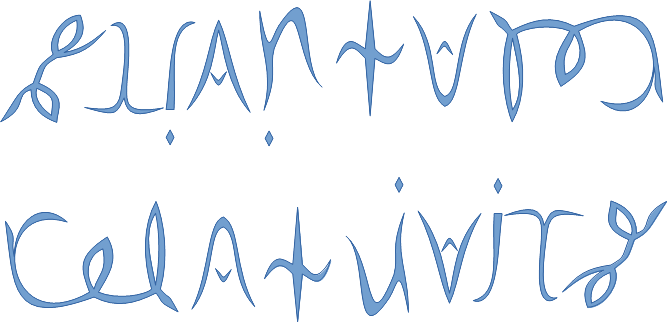See SRQM: QM from SR - The 4-Vector RoadMap (.html)
See SRQM: QM from SR - The 4-Vector RoadMap (.pdf)

SRQM Physics Diagramming Method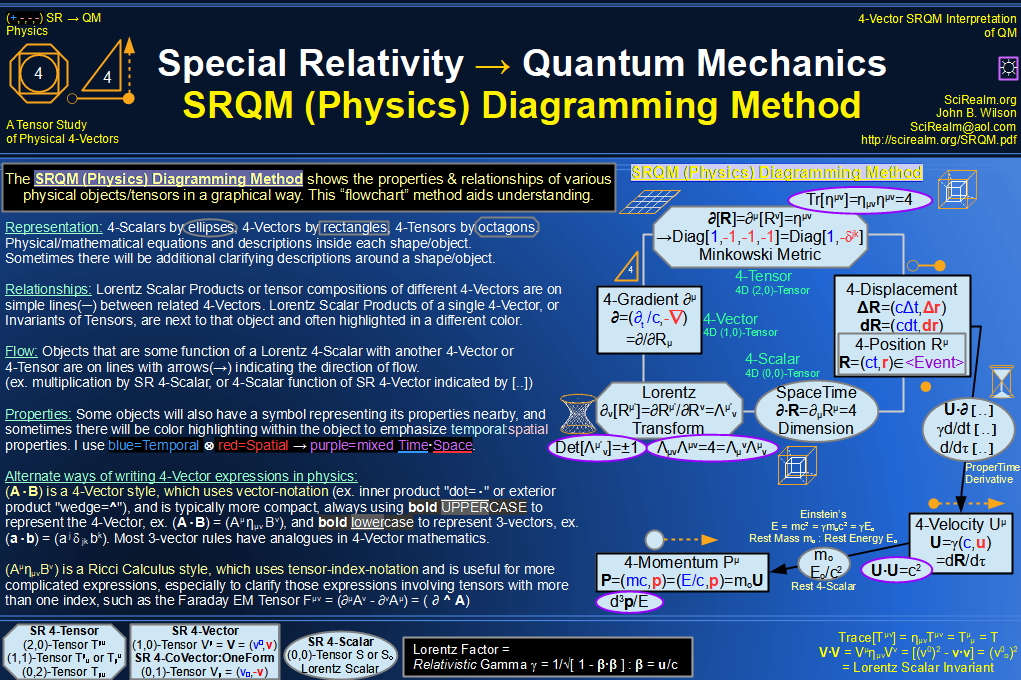SRQM 4-Vector : Four-Vector and Lorentz Scalar Diagram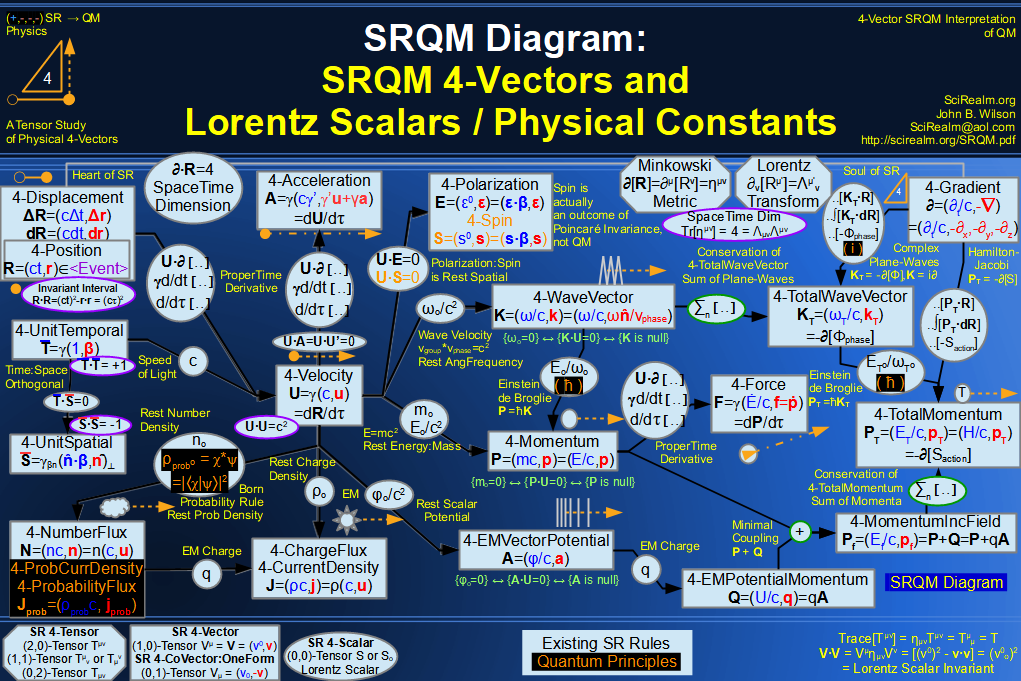SRQM + EM 4-Vector : Four-Vector and Lorentz Scalar Diagram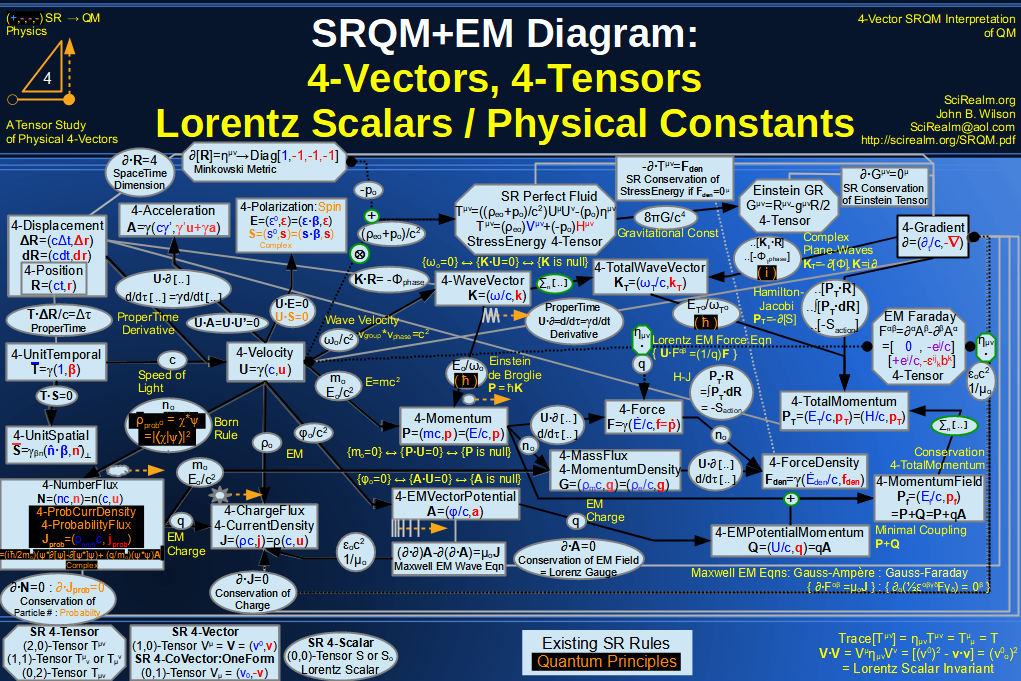SRQM + EM 4-Vector : Four-Vector and Lorentz Scalar Diagram With Tensor Invariants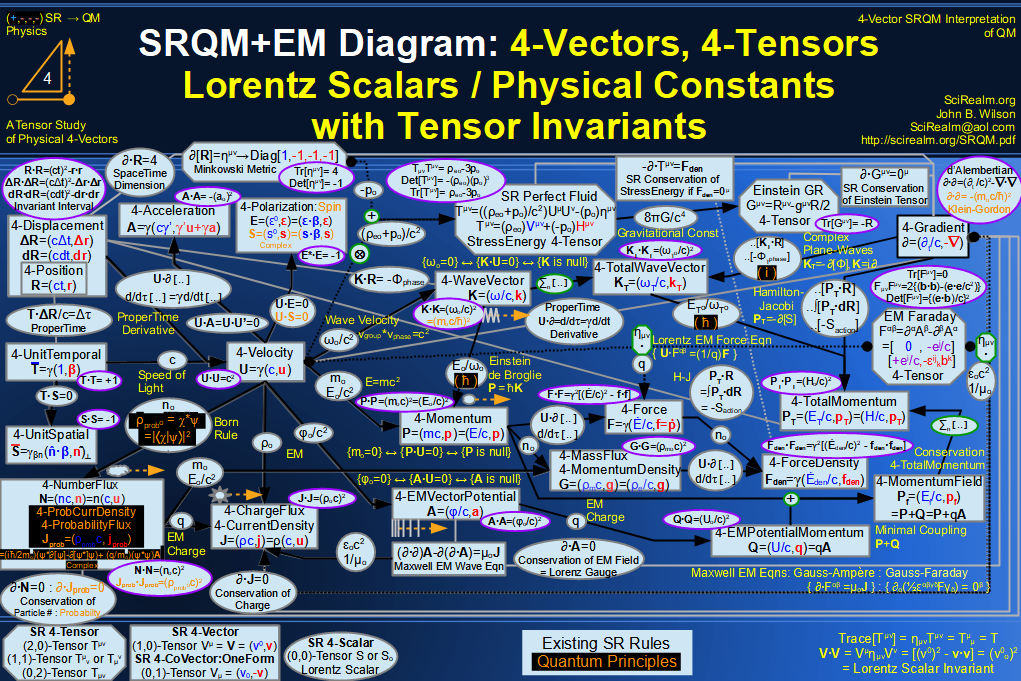SRQM 4-Vector : Four-Vector Stress-Energy & Projection Tensors Diagram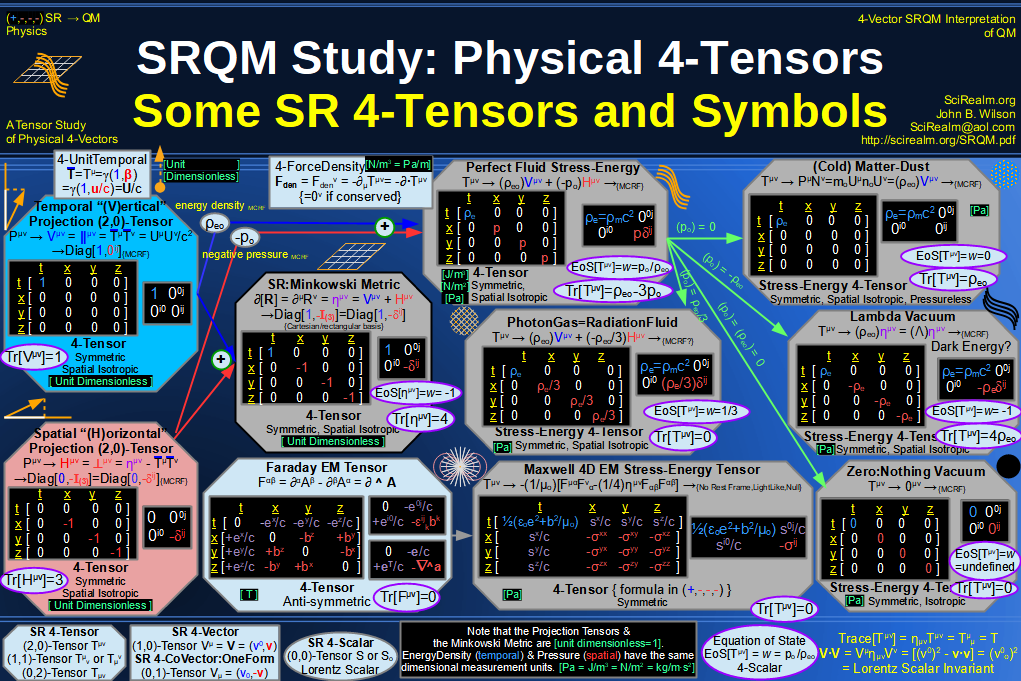SRQM 4-Vector : Four-Vector SR Quantum RoadMap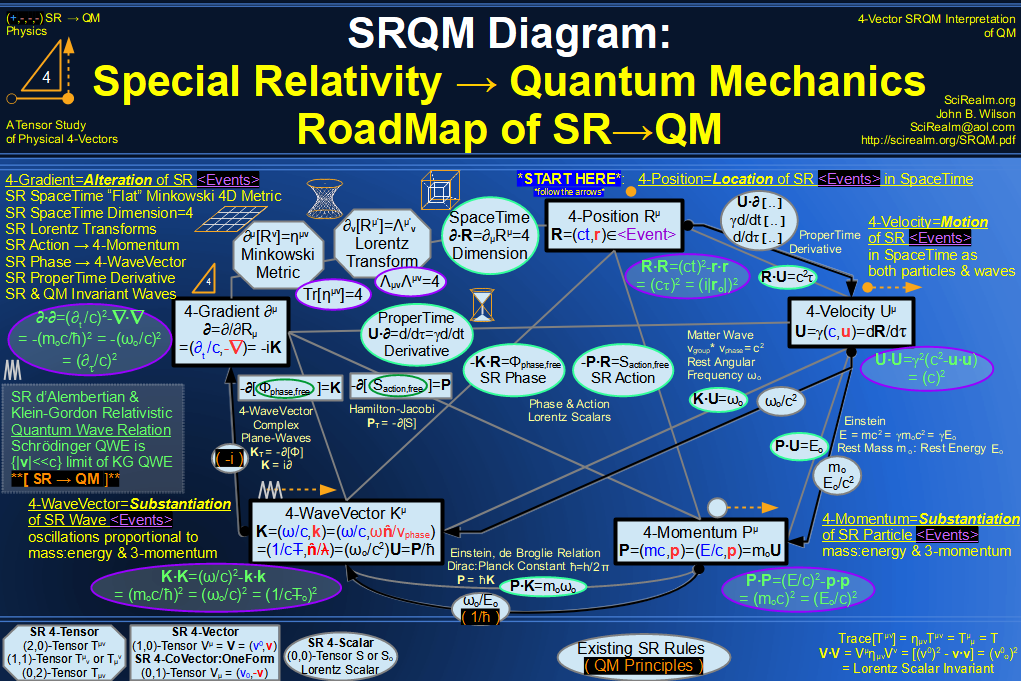SRQM + EM 4-Vector : Four-Vector SR Quantum RoadMap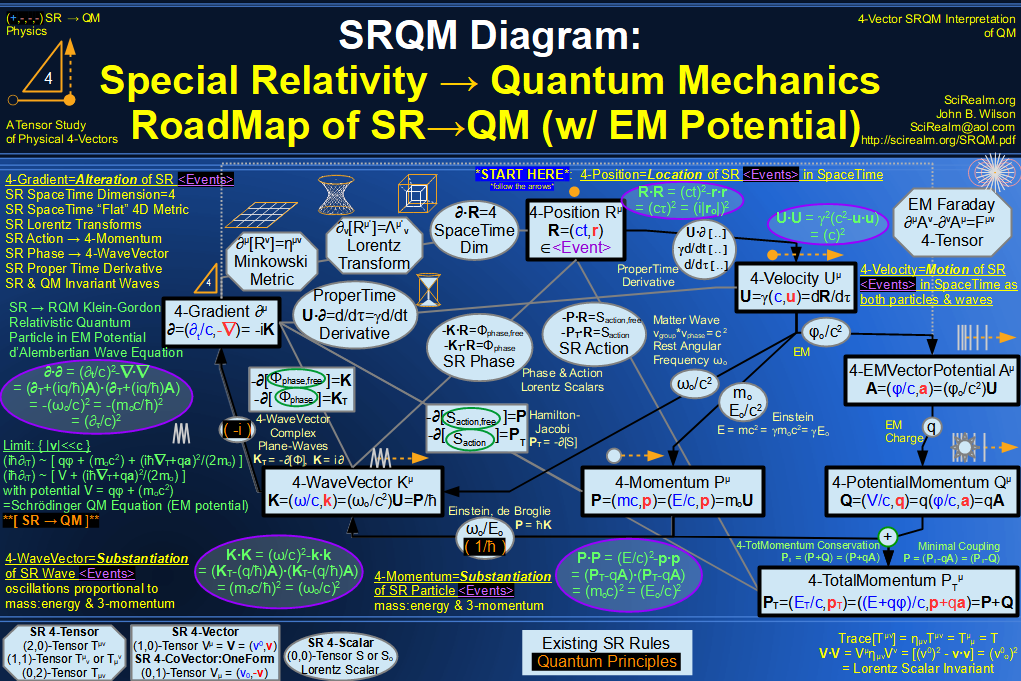SRQM + EM 4-Vector : Four-Vector SR Quantum RoadMap - Simple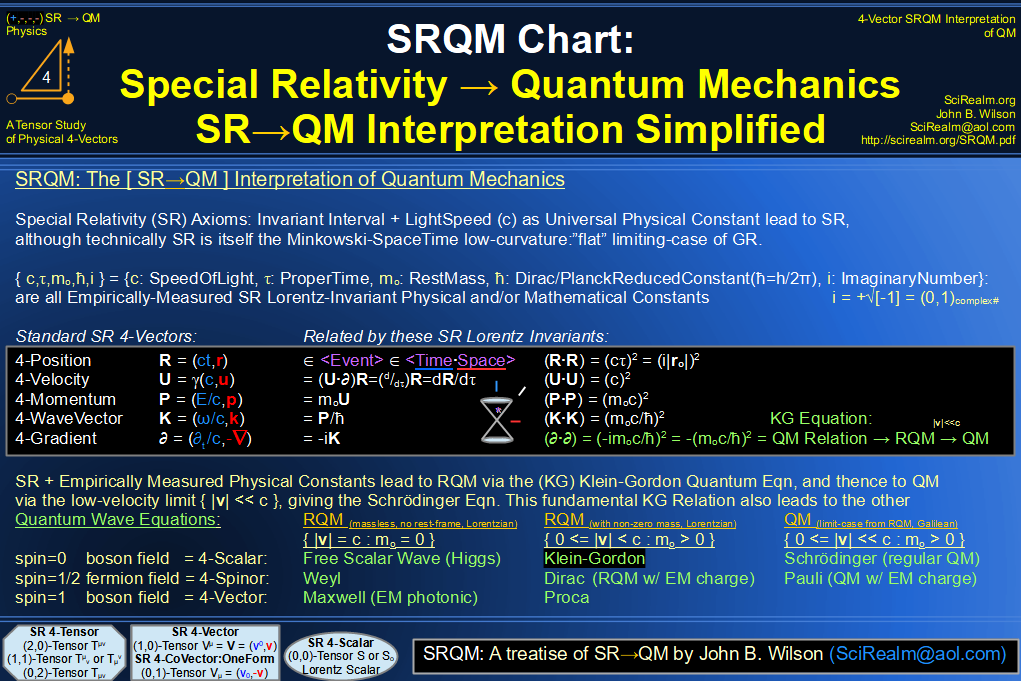SRQM 4-Vector : Four-Vector New Relativistic Quantum Paradigm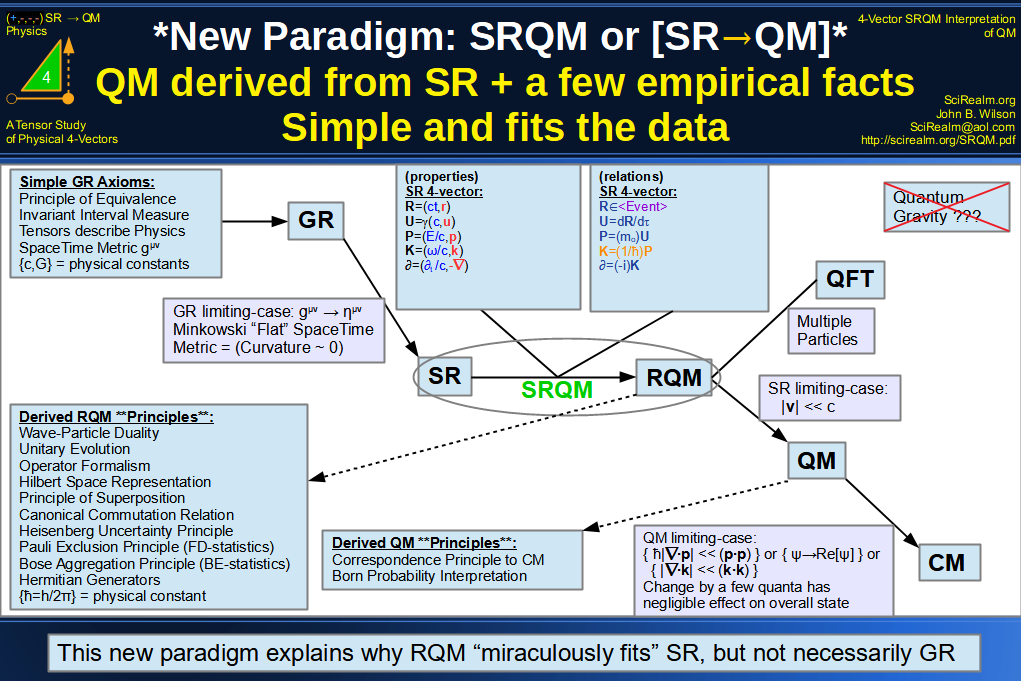SRQM + EM 4-Vector : Four-Vector New Relativistic Quantum Paradigm (with EM)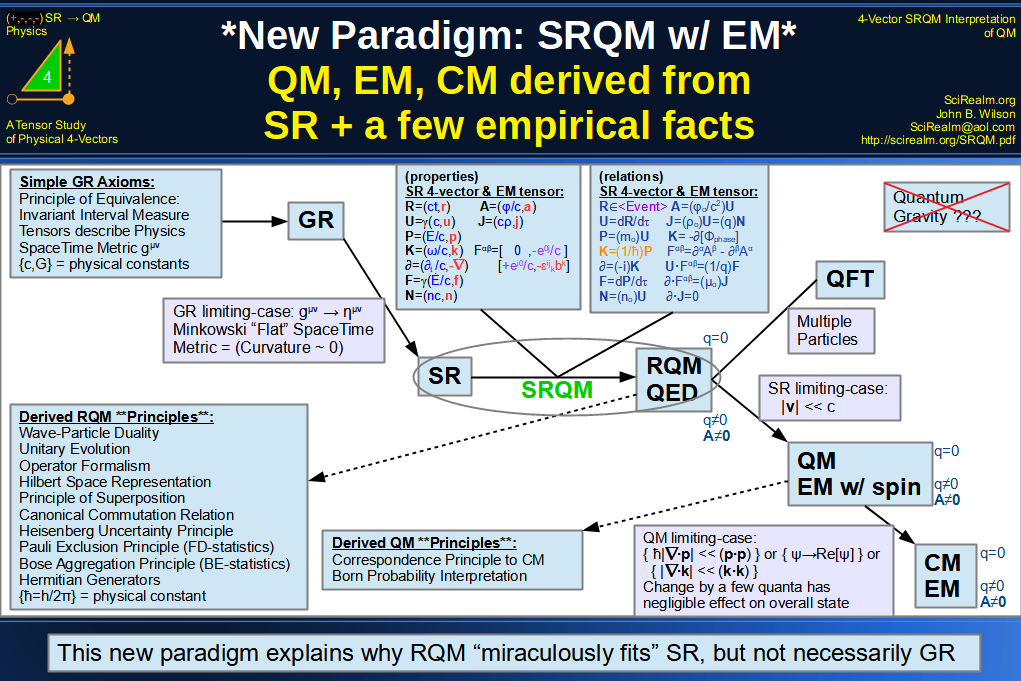SRQM 4-Vector : Four-Vector New Relativistic Quantum Paradigm - Venn Diagram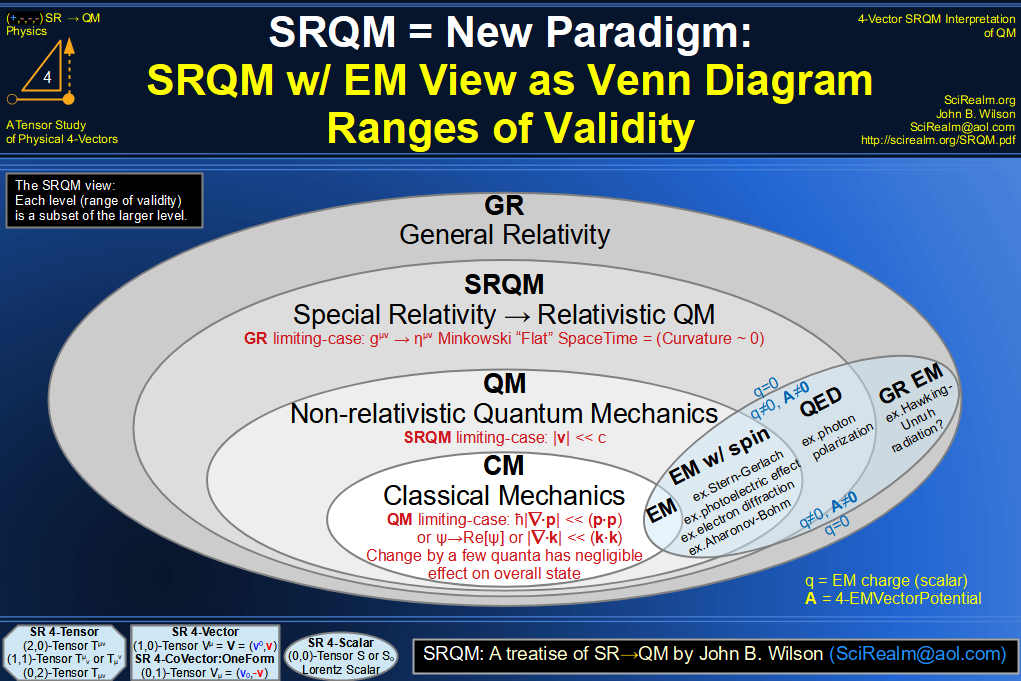SRQM 4-Vector : Four-Vector SpaceTime is 4D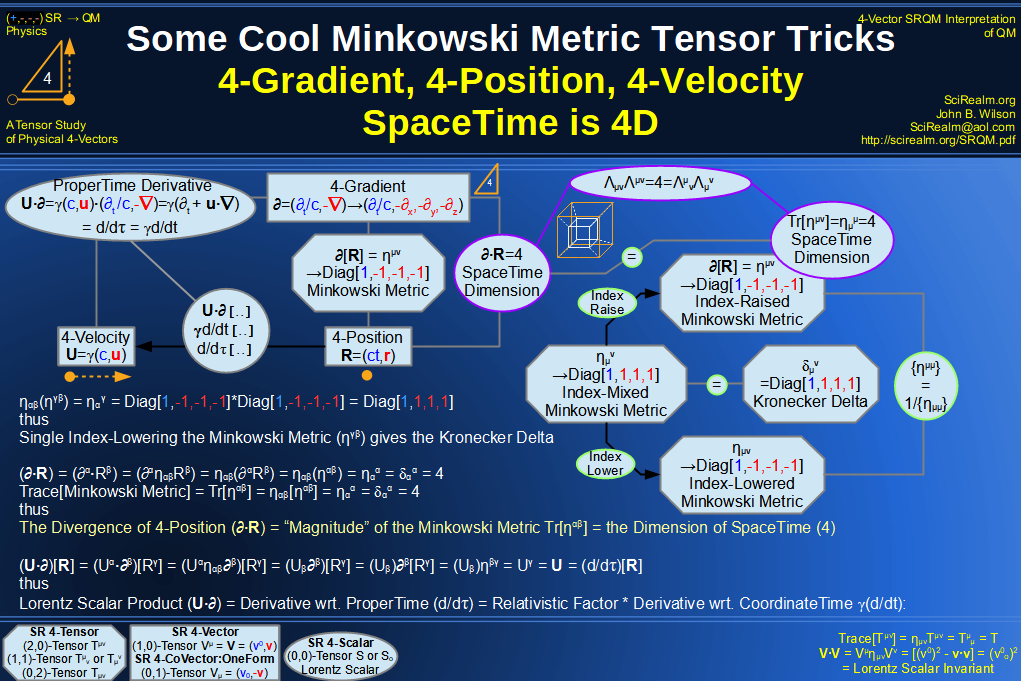SRQM 4-Vector : Four-Vector SpaceTime Orthogonality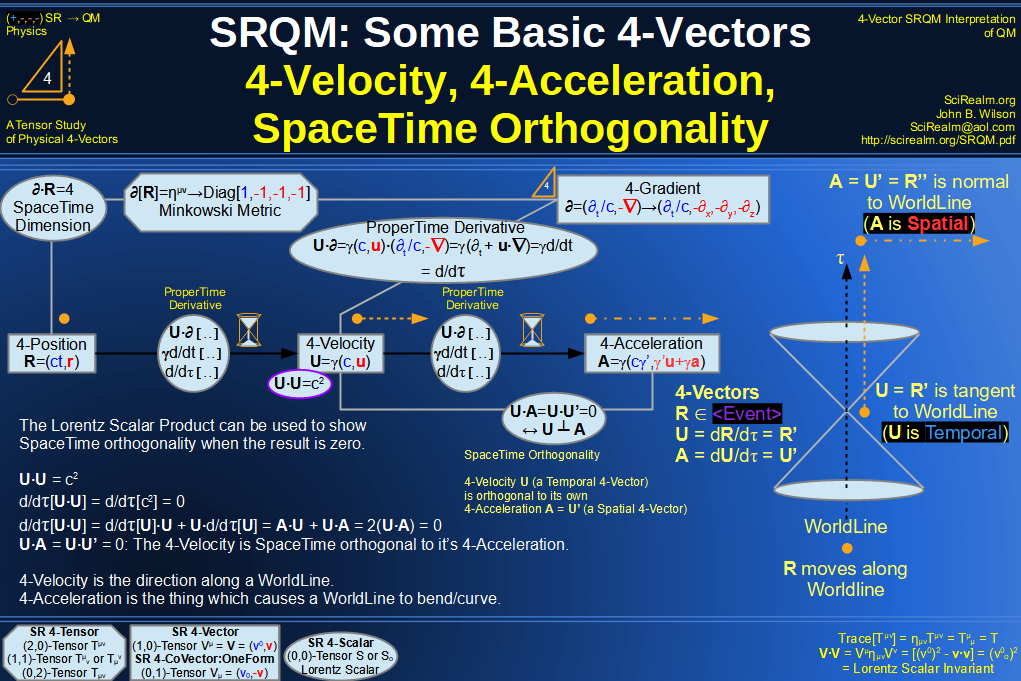SRQM 4-Vector : Four-Vector 4-Position, 4-Velocity, 4-Acceleration Diagram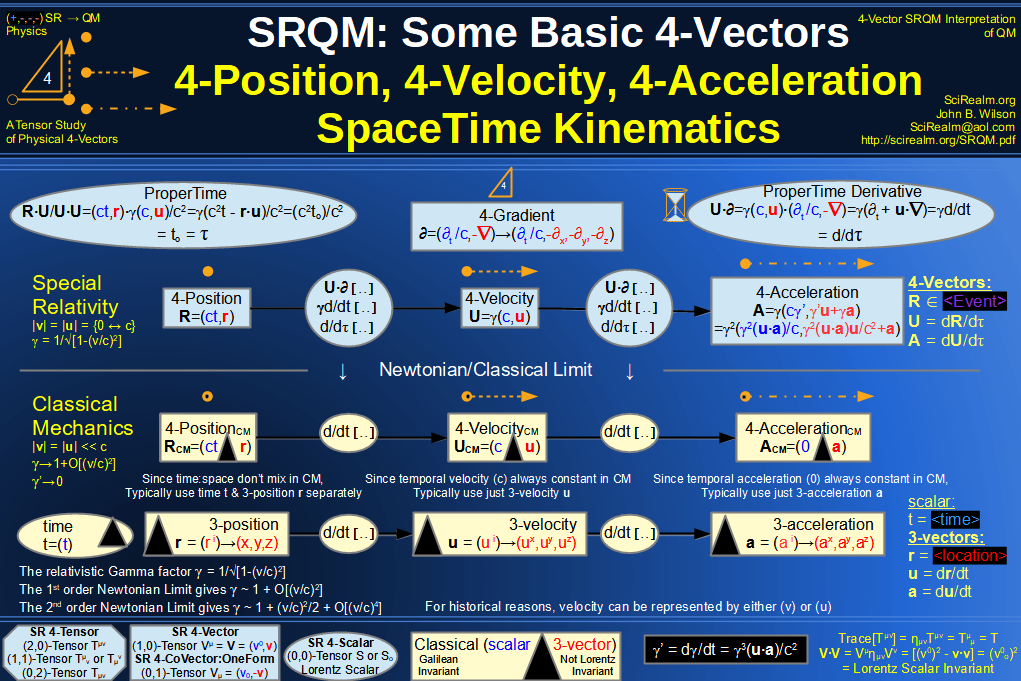SRQM 4-Vector : Four-Vector 4-Displacement, 4-Velocity, Relativity of Simultaneity Diagram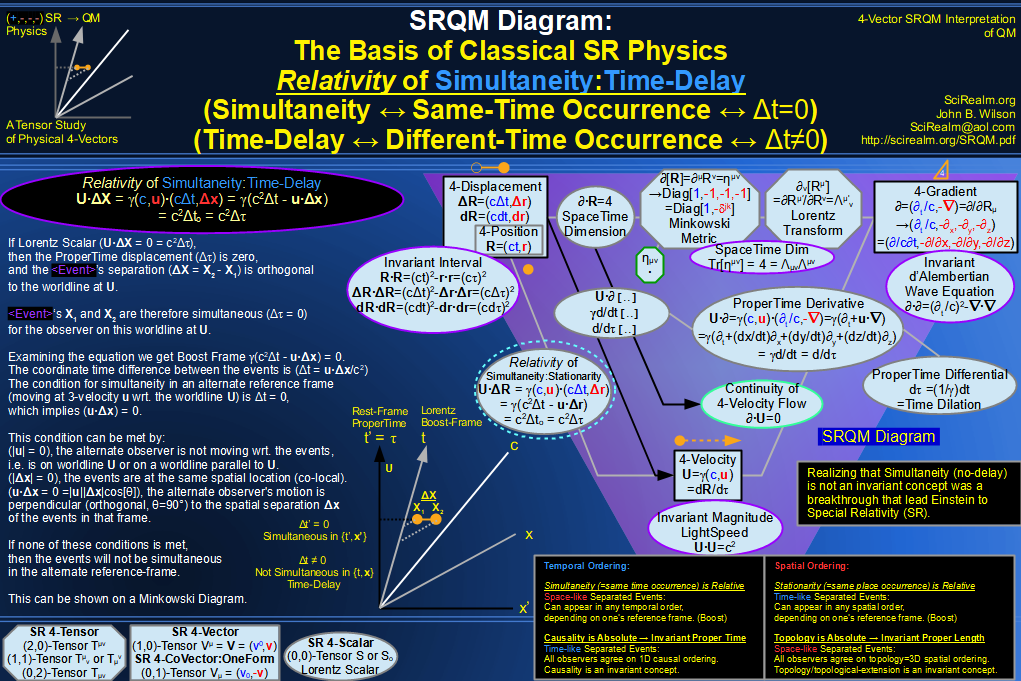SRQM 4-Vector : Four-Vector 4-Velocity, 4-Gradient, Time Dilation Diagram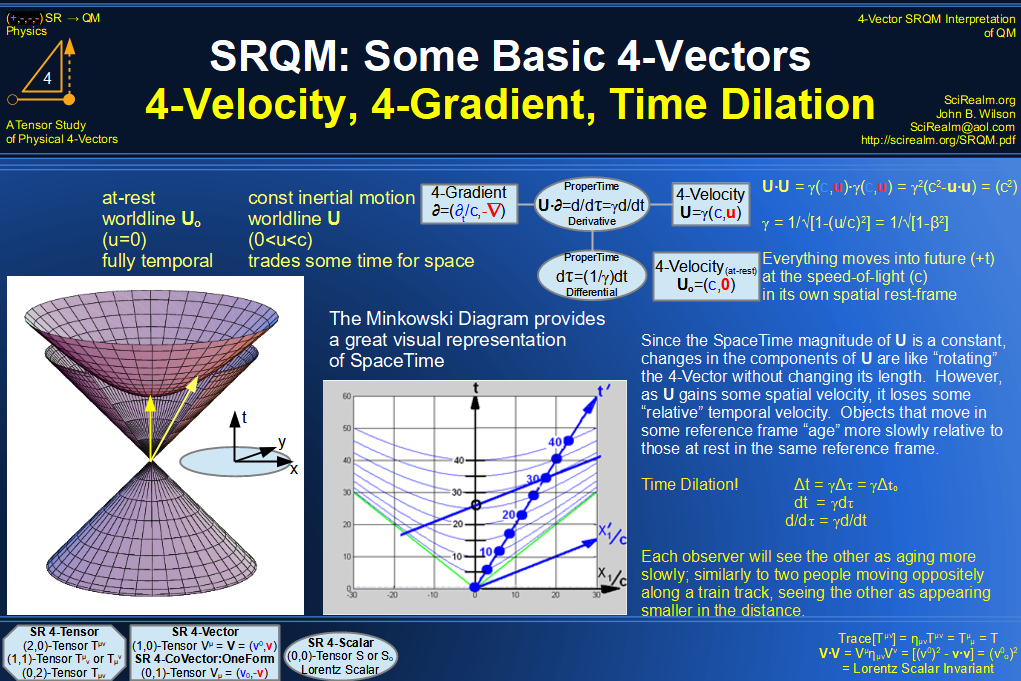SRQM 4-Vector : Four-Vector 4-Vector, 4-Velocity, 4-Momentum, E=mc^2 Diagram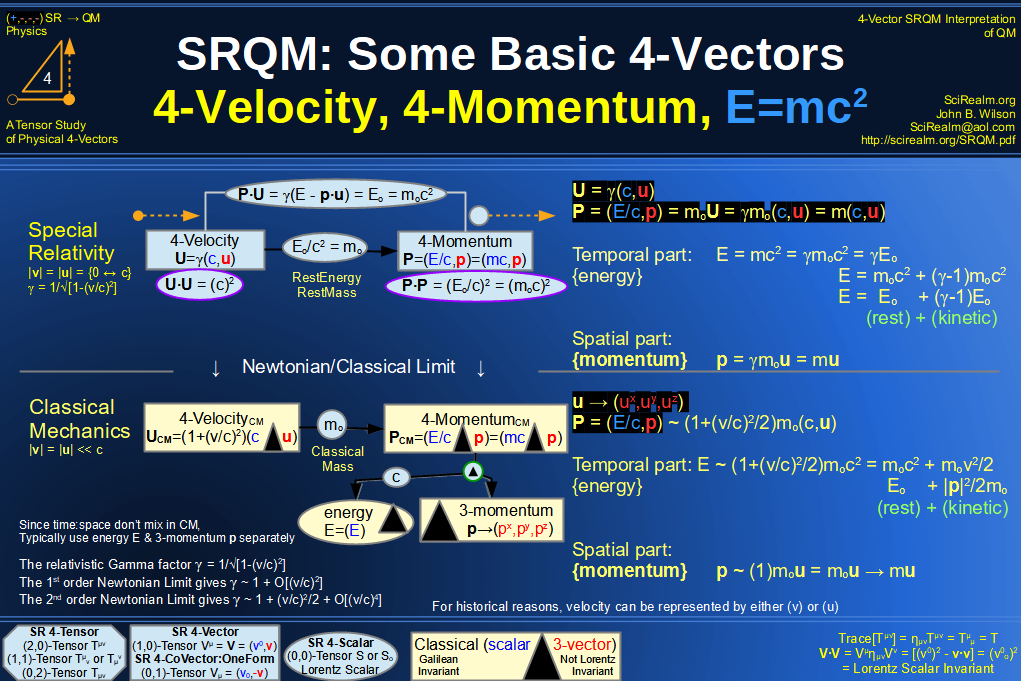SRQM 4-Vector : Four-Vector 4-Velocity, 4-WaveVector, Relativistic Doppler Effect Diagram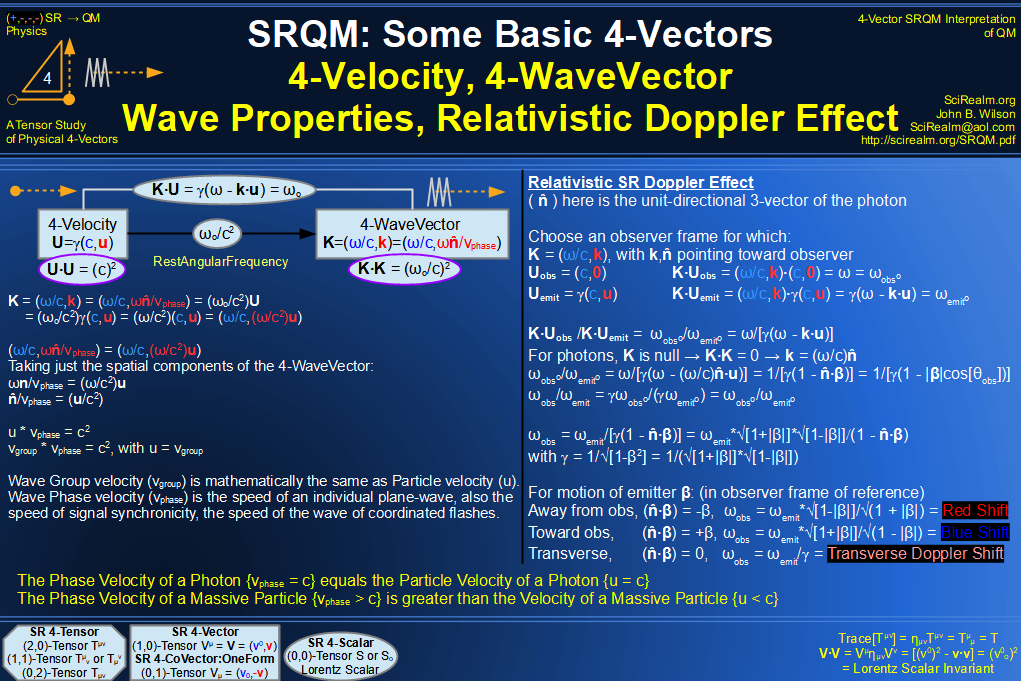SRQM 4-Vector : Four-Vector Wave-Particle Diagram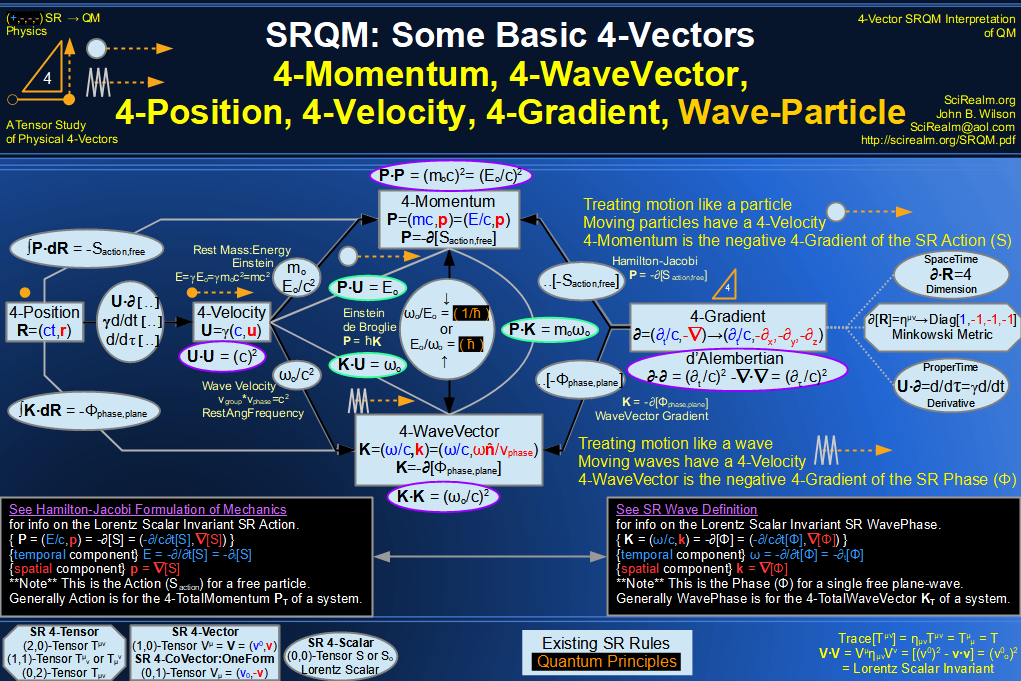SRQM 4-Vector : Four-Vector Compton Effect Diagram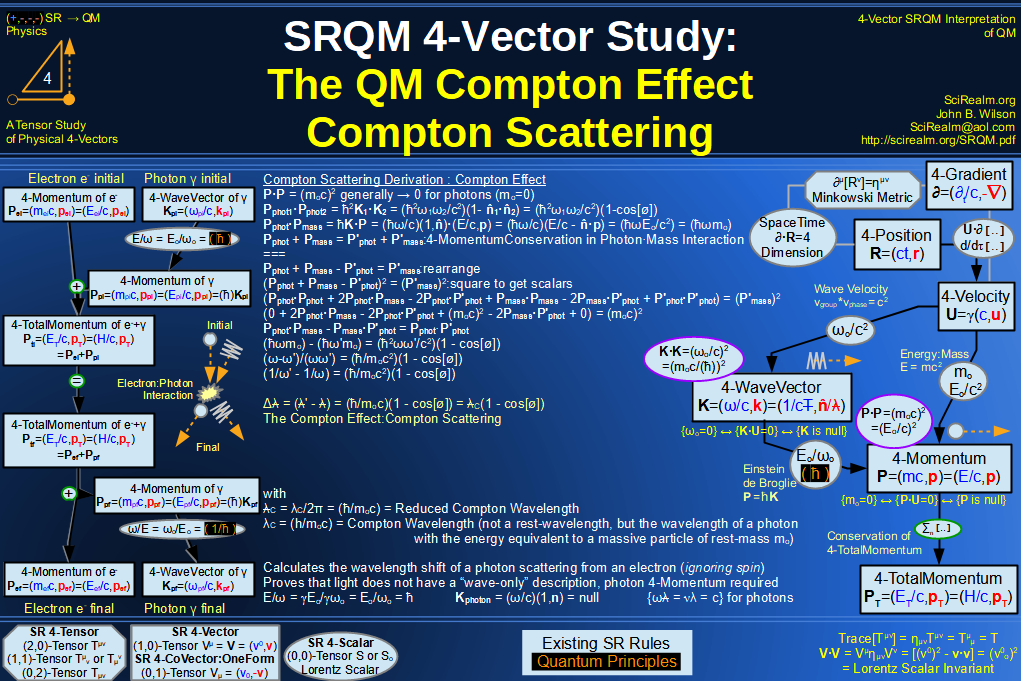SRQM 4-Vector : Four-Vector Aharonov-Bohm Effect Diagram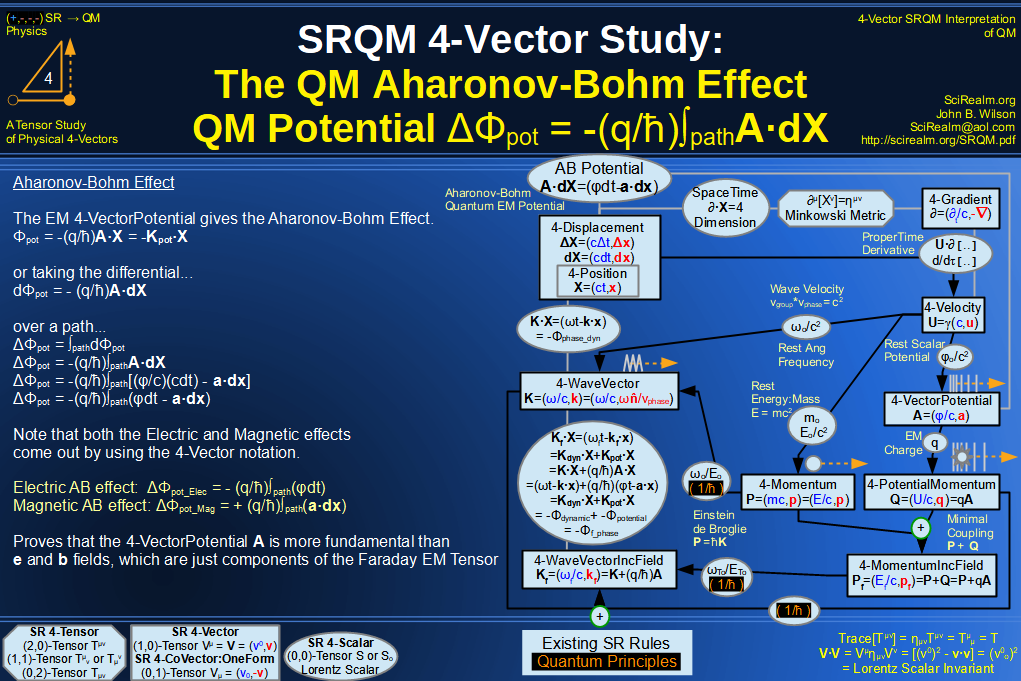SRQM 4-Vector : Four-Vector Josephson Junction Effect Diagram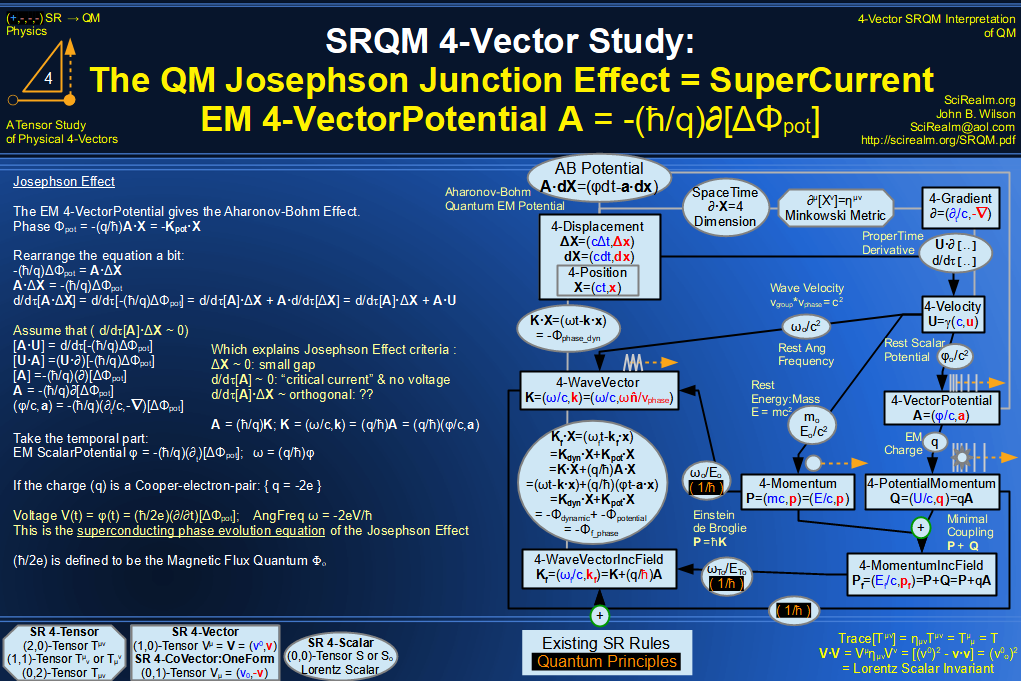SRQM 4-Vector : Four-Vector Hamilton-Jacobi vs Action, Josephson vs Aharonov-Bohm Diagram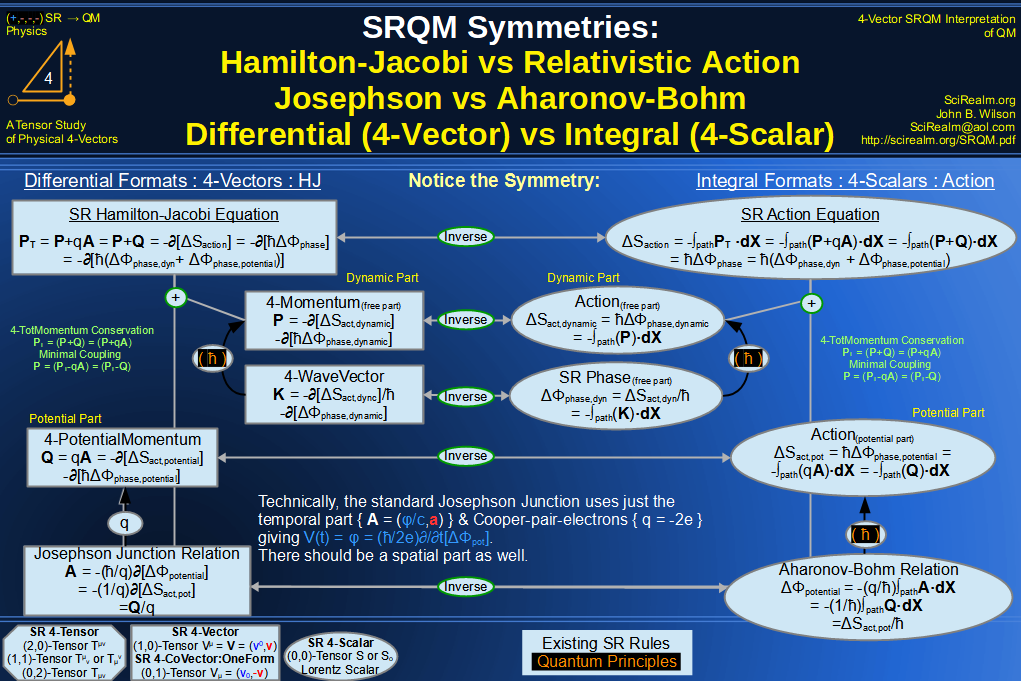SRQM 4-Vector : Four-Vector Motion of Lorentz Scalar Invariants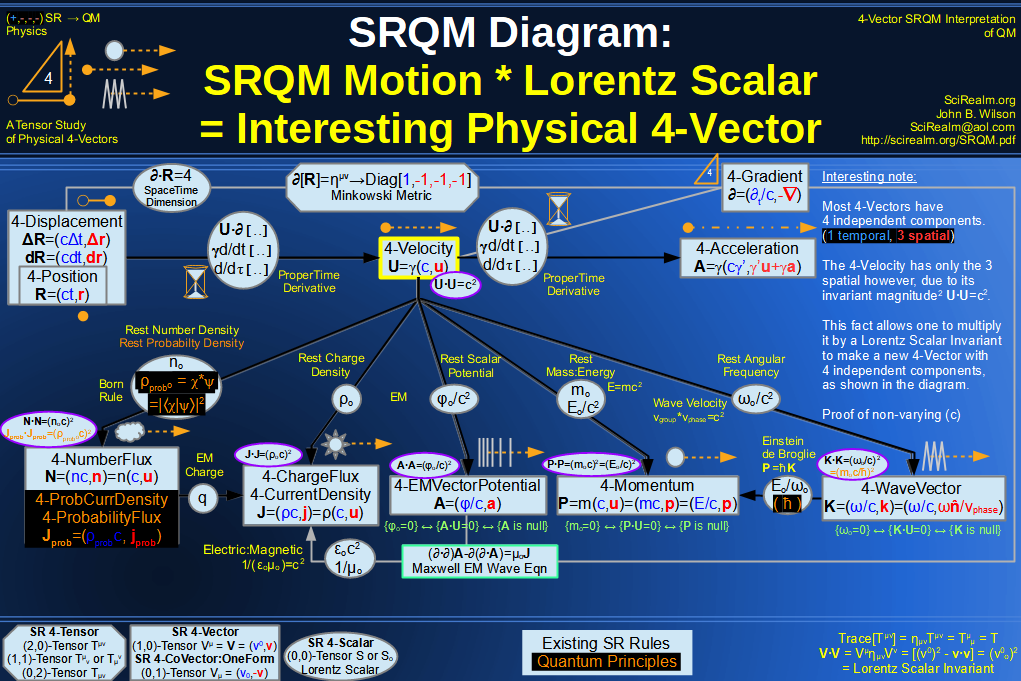SRQM 4-Vector : Four-Vector Motion of Lorentz Scalar Invariants, Conservation Laws & Continuity Equations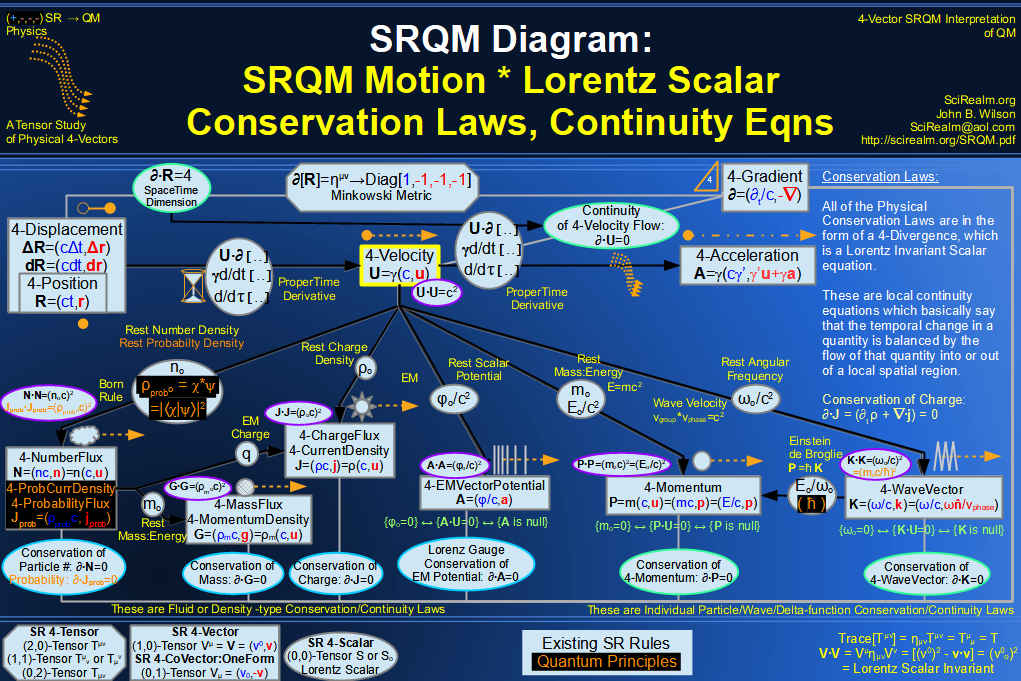SRQM 4-Vector : Four-Vector Speed of Light (c)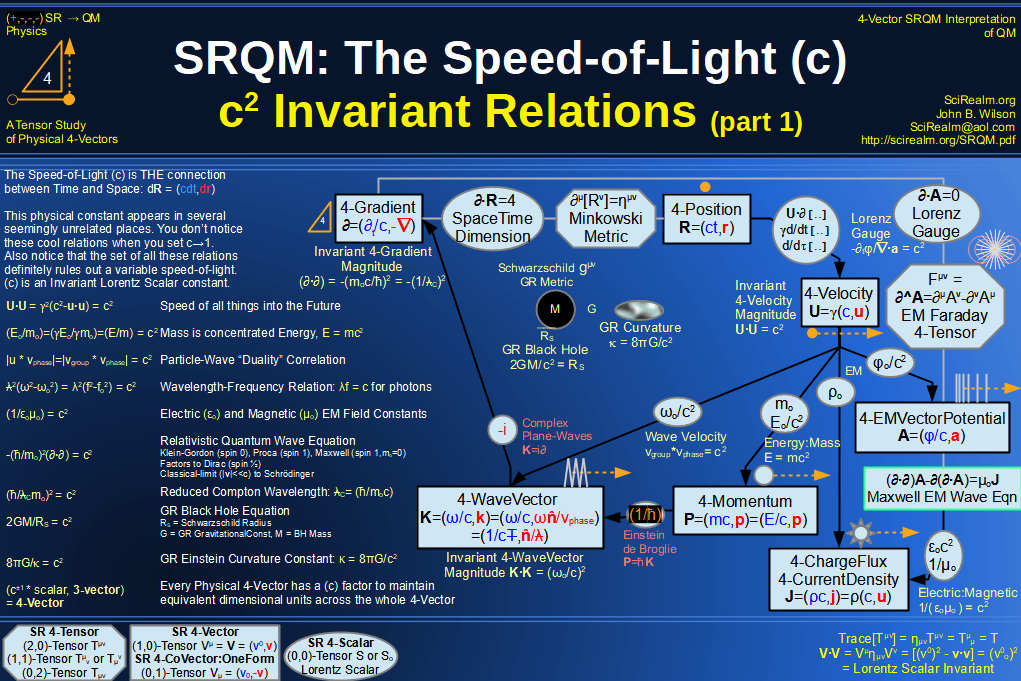SRQM 4-Vector : Four-Vector Minimal Coupling Conservation of 4-TotalMomentum)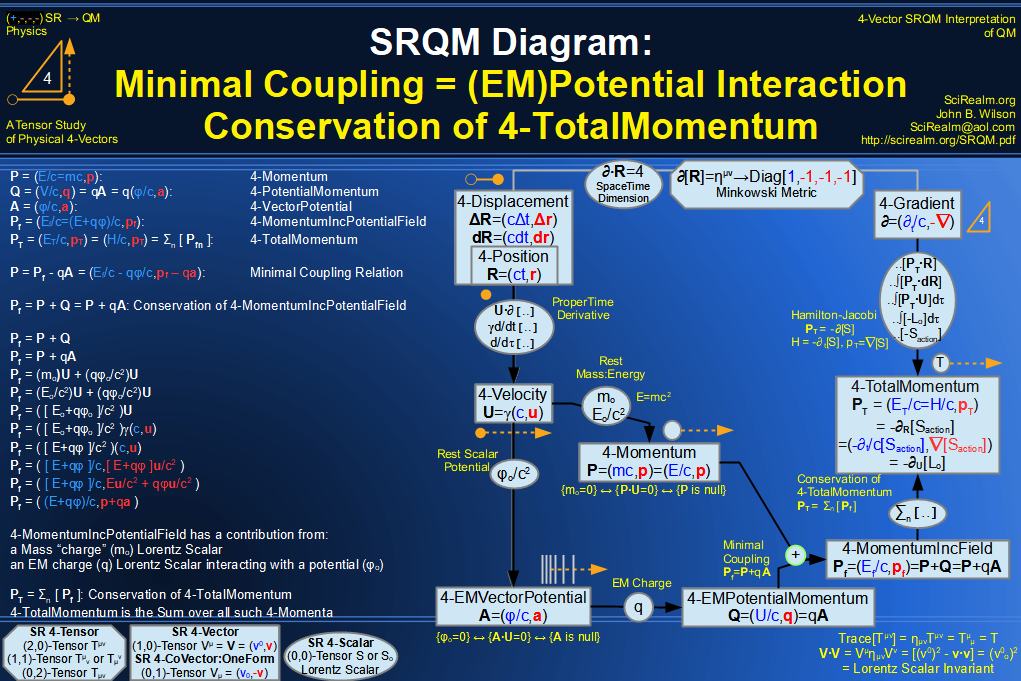SRQM 4-Vector : Four-Vector Relativistic Action (S) Diagram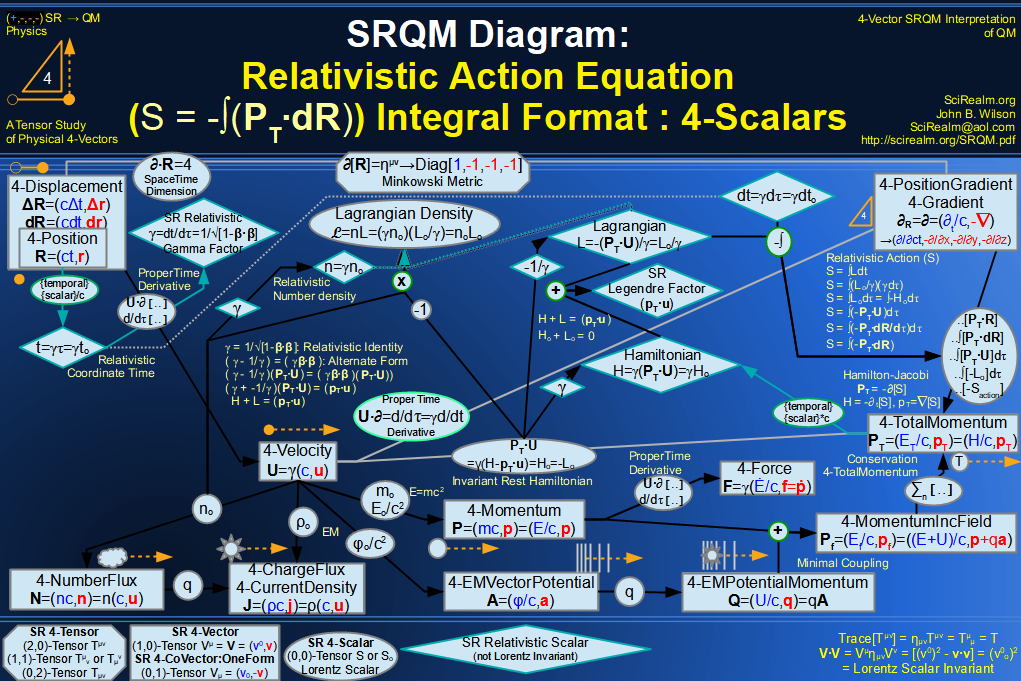SRQM 4-Vector : Four-Vector Relativistic Lagrangian Hamiltonian Diagram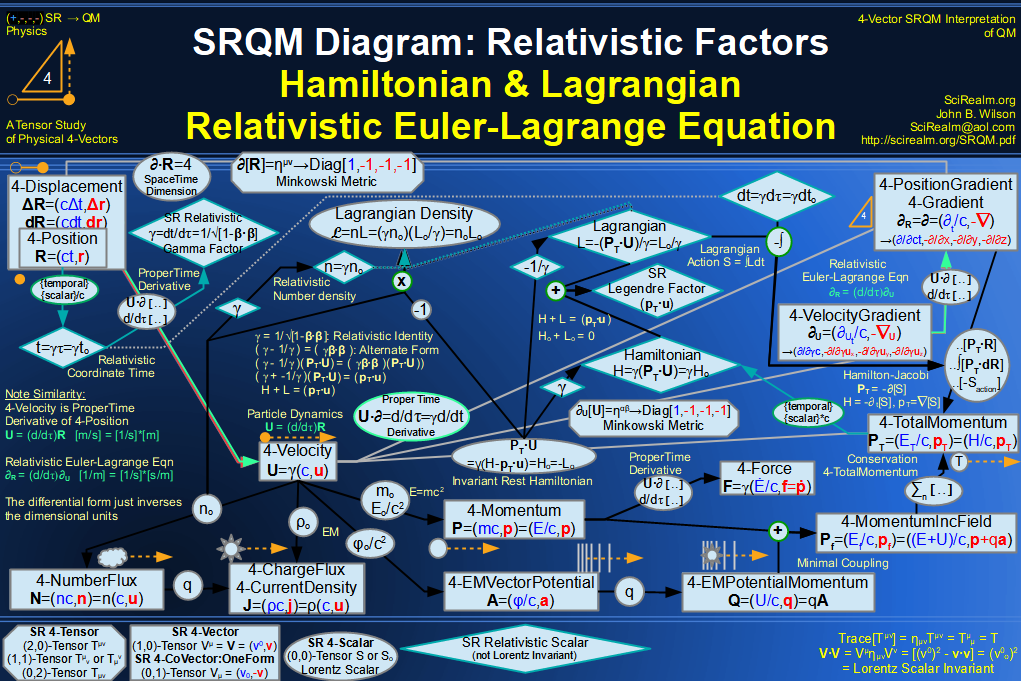SRQM 4-Vector : Four-Vector Relativistic Euler-Lagrange Equation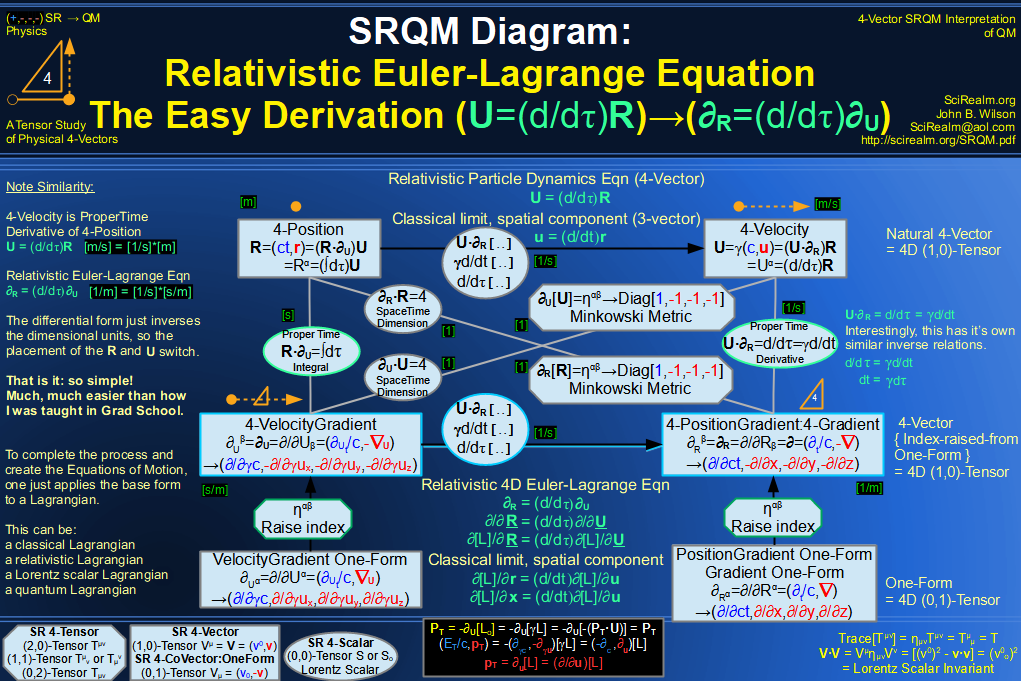SRQM 4-Vector : Four-Vector Relativistic EM Equations of Motion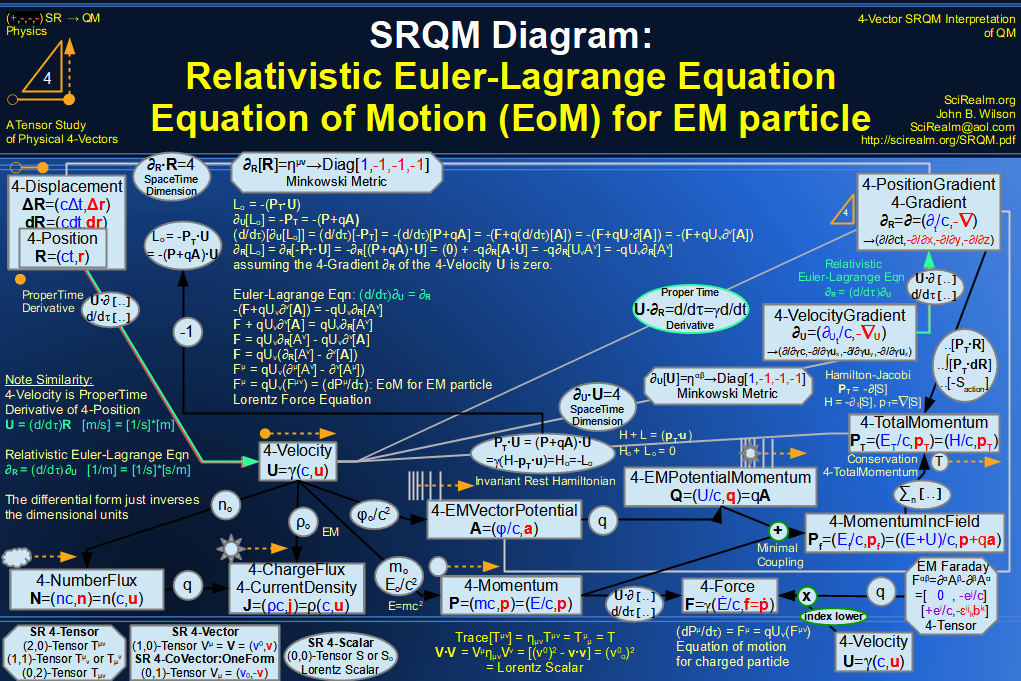SRQM 4-Vector : Four-Vector Einstein-de Broglie Relation hbar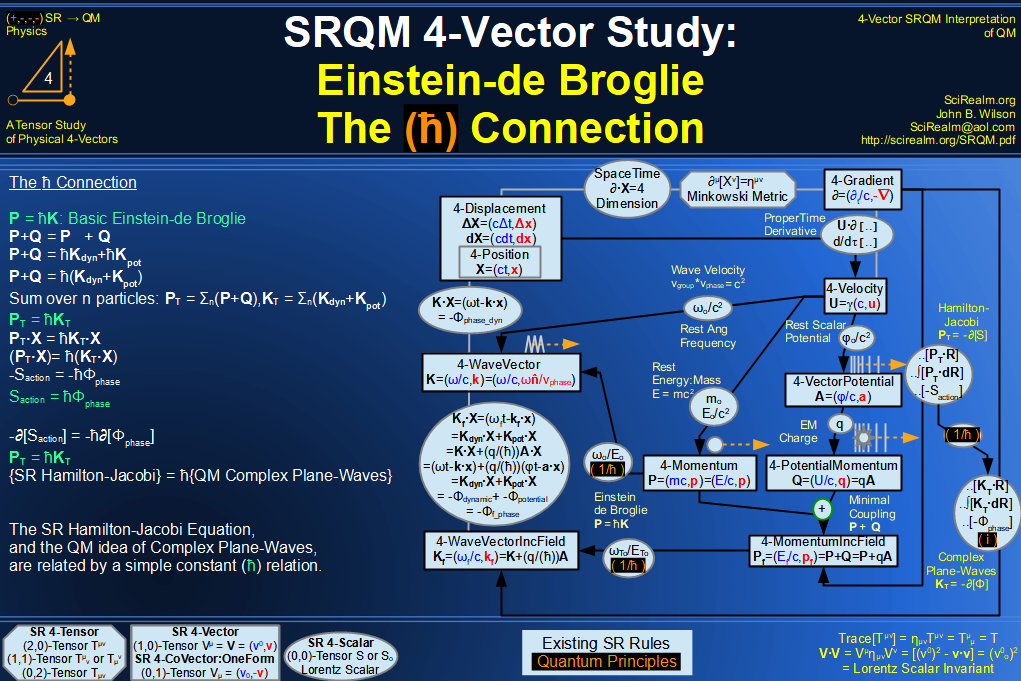SRQM 4-Vector : Four-Vector Quantum Canonical Commutation Relation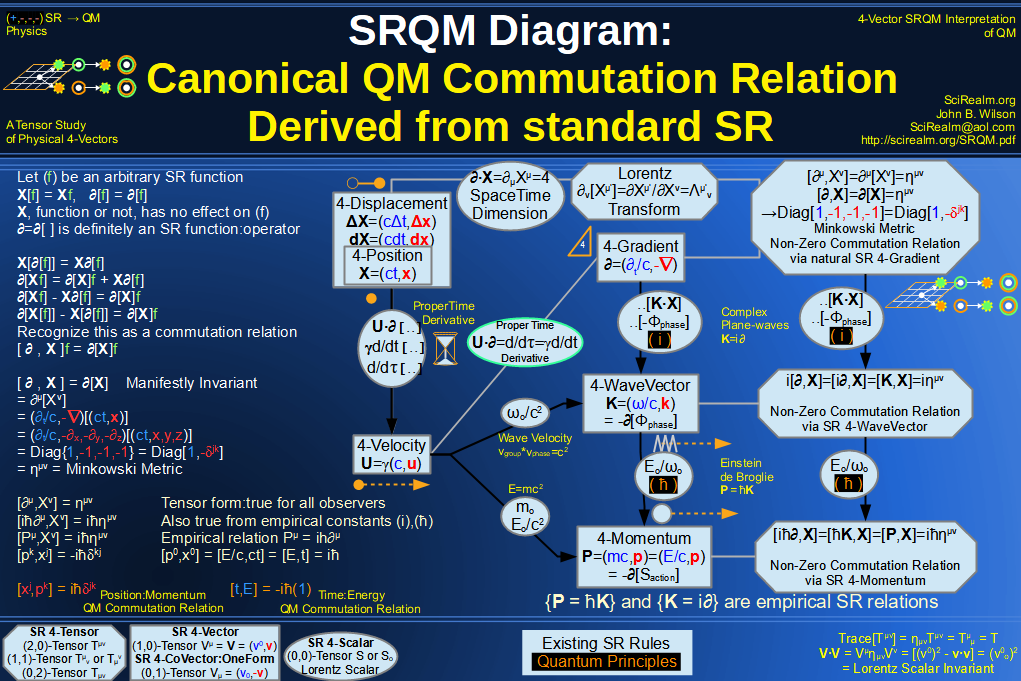SRQM 4-Vector : Four-Vector QM Schroedinger Relation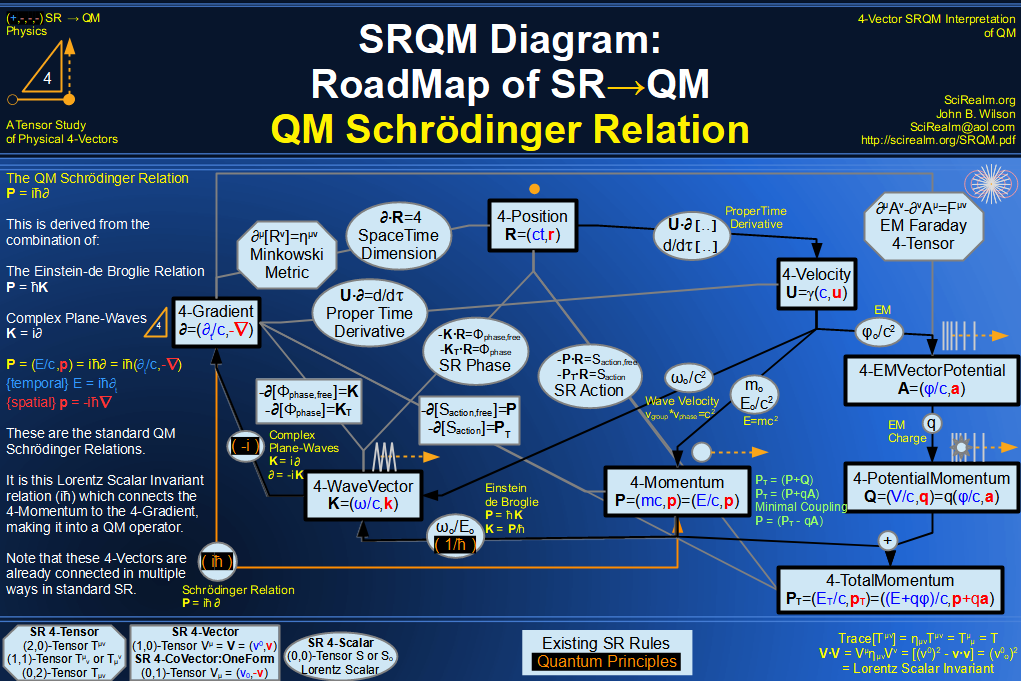SRQM 4-Vector : Four-Vector Quantum Probability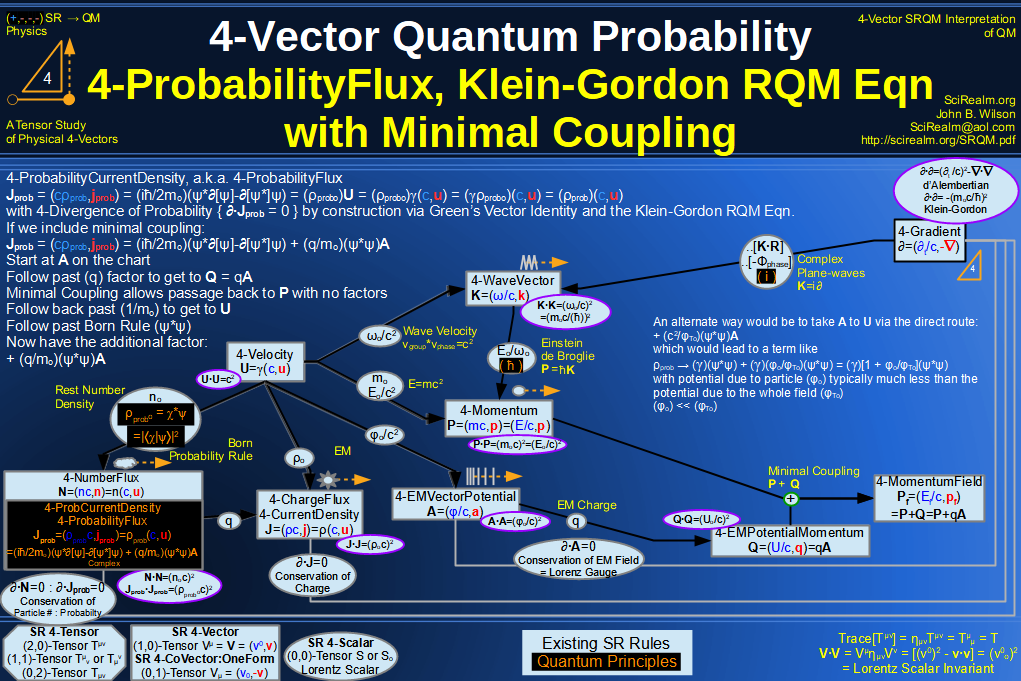SRQM 4-Vector : Four-Vector CPT Theorem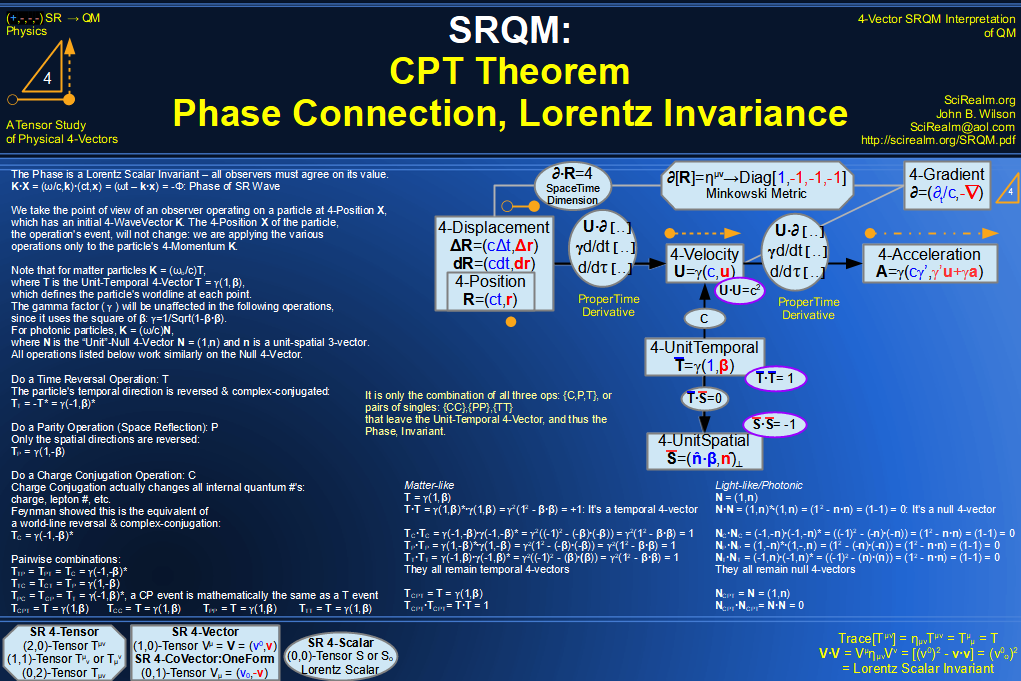SRQM 4-Vector : Four-Vector Lorentz Transforms Connection Map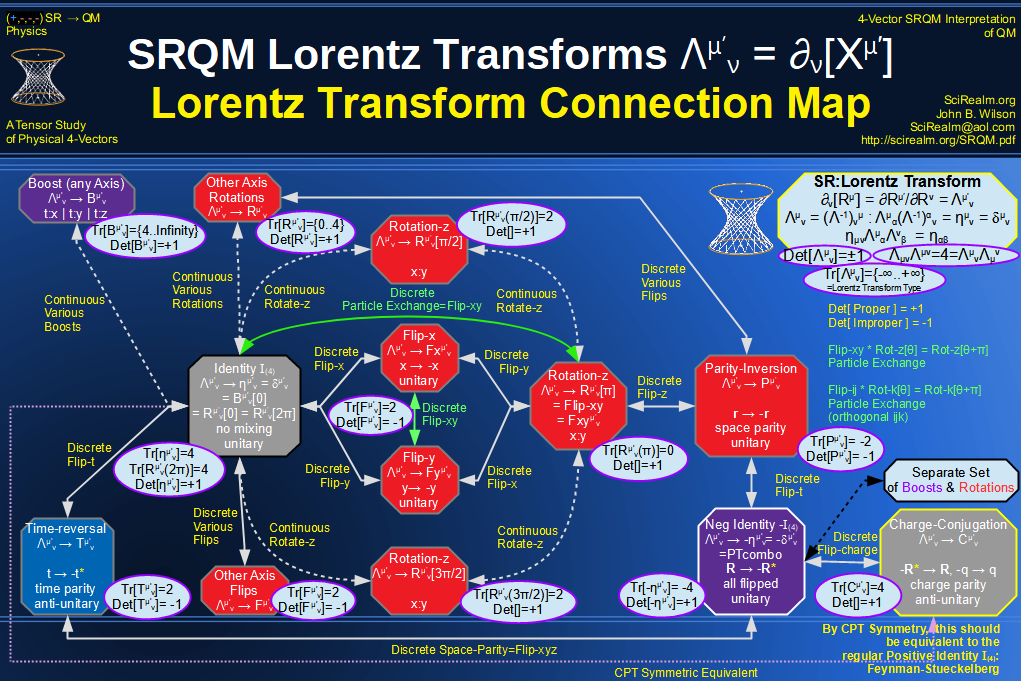SRQM 4-Vector : Four-Vector Lorentz Discrete Transforms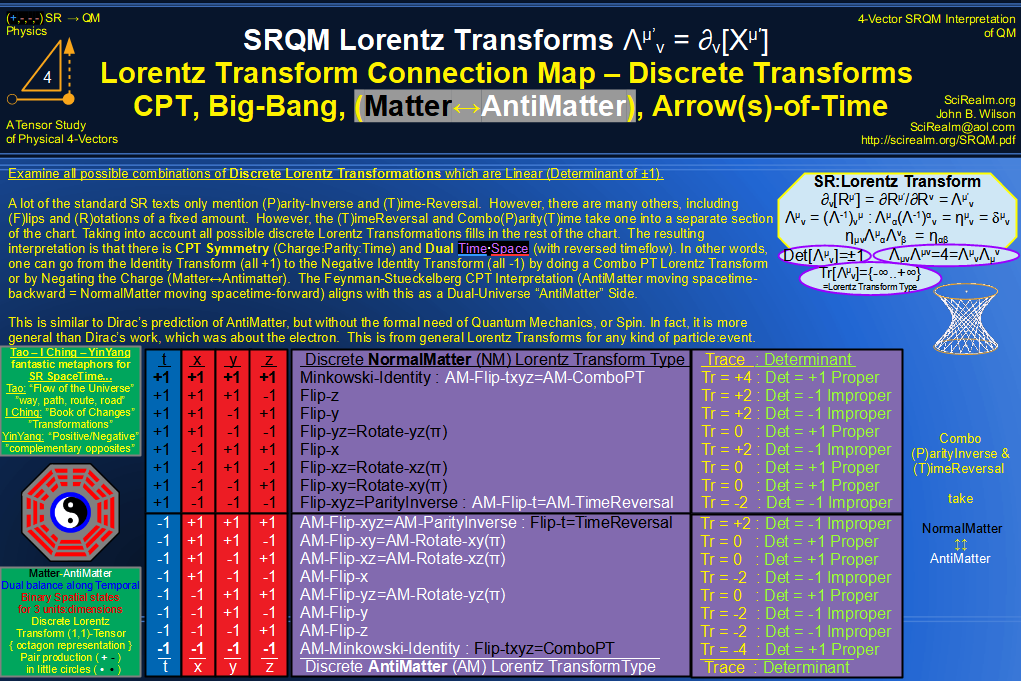SRQM 4-Vector : Four-Vector Lorentz Transforms - Trace Identification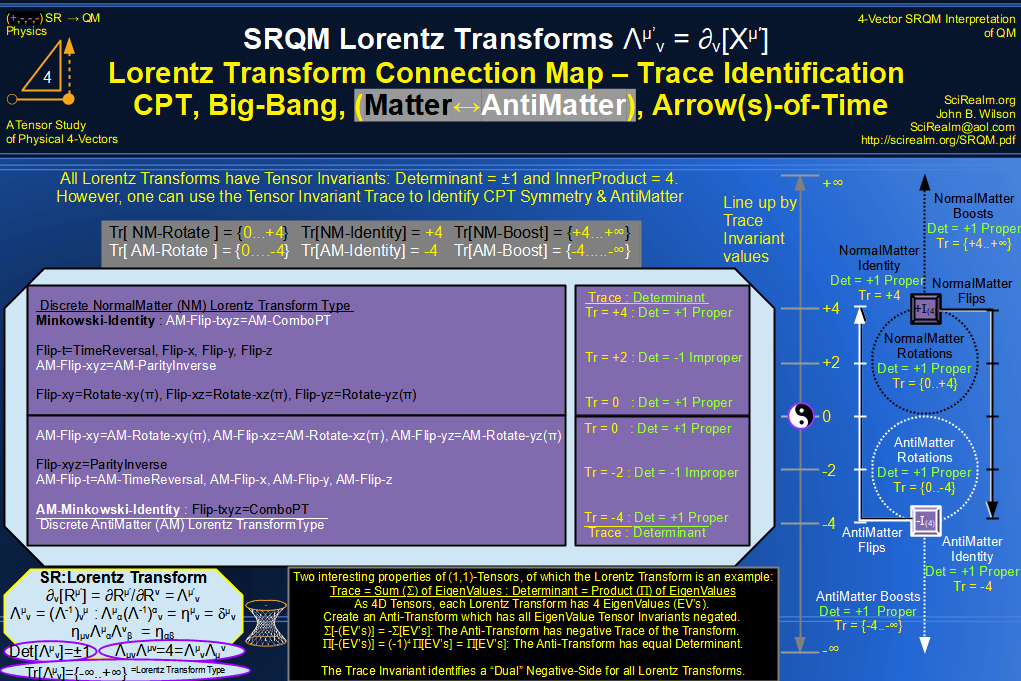SRQM 4-Vector : Four-Vector Lorentz Lorentz Transforms-Interpretations
CPT Symmetry, Baryon Asymmetry Problem Solution, Matter-Antimatter Symmetry Solution, Arrow-of-Time Problem Solution, Big-Bang!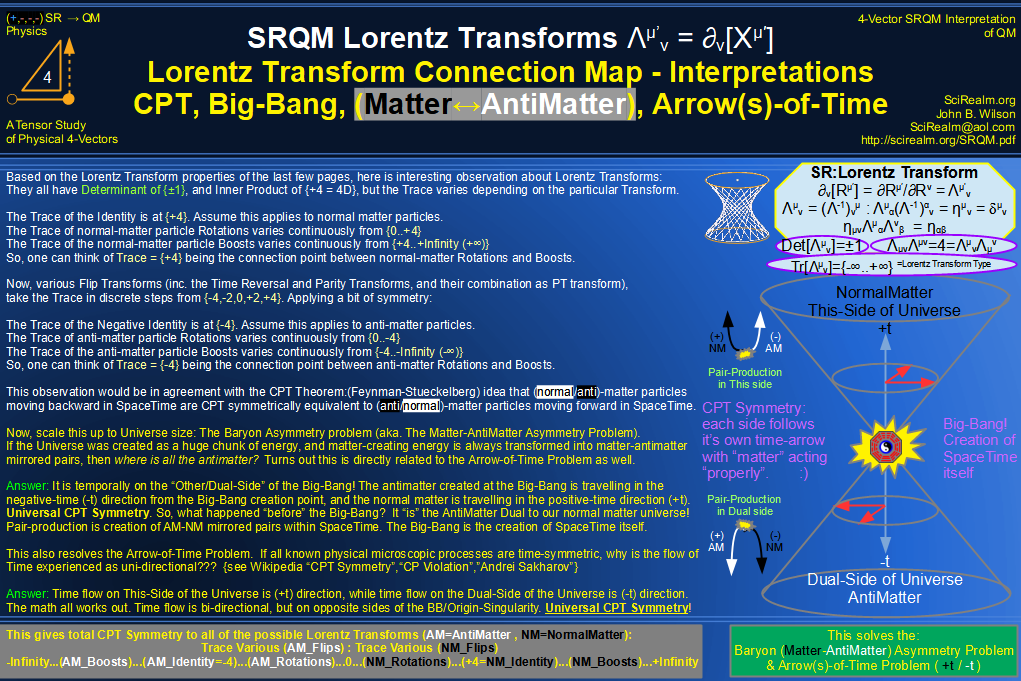See SRQM: QM from SR - The 4-Vector RoadMap (.html)
See SRQM: QM from SR - The 4-Vector RoadMap (.pdf)The Science Realm: John's Virtual Sci-Tech Universe John's Science & Math Stuff: | About John | Send email to John 4-Vectors | Ambigrams | Antipodes | Covert Ops Fragments | Cyrillic Projector | Forced Induction (Sums Of Powers Of Integers) | Fractals | Frontiers | JavaScript Graphics | Kid Science | Kryptos | Philosophy | Photography | Prime Sieve | QM from SR | QM from SR-Simple RoadMap | SR 4-Vector & Tensor Calculator | Quantum Phase | Quotes | RuneQuest Cipher Challenge | Scientific Calculator (complex capable) | Secret Codes & Ciphers | Science+Math | Sci-Pan Pantheist Poems | Stereograms | SuperMagicSqr4x4 | Turkish Grammar | Quantum Mechanics is derivable from Special Relativity See SRQM - QM from SR - Simple RoadMap (.html) See SRQM - QM from SR - Simple RoadMap (.pdf)